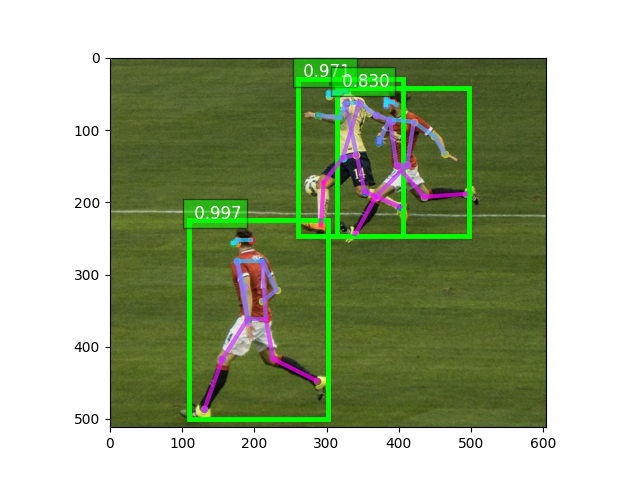# 1. Predict with pre-trained Simple Pose Estimation models¶

This article shows how to play with pre-trained Simple Pose models with only a few lines of code.

First let’s import some necessary libraries:

from matplotlib import pyplot as plt
from gluoncv import model_zoo, data, utils
from gluoncv.data.transforms.pose import detector_to_simple_pose, heatmap_to_coord


Let’s get a Simple Pose model trained with input images of size 256x192 on MS COCO dataset. We pick the one using ResNet-18 V1b as the base model. By specifying pretrained=True, it will automatically download the model from the model zoo if necessary. For more pretrained models, please refer to Model Zoo.

Note that a Simple Pose model takes a top-down strategy to estimate human pose in detected bounding boxes from an object detection model.

detector = model_zoo.get_model('yolo3_mobilenet1.0_coco', pretrained=True)
pose_net = model_zoo.get_model('simple_pose_resnet18_v1b', pretrained=True)

# Note that we can reset the classes of the detector to only include
# human, so that the NMS process is faster.

detector.reset_class(["person"], reuse_weights=['person'])


## Pre-process an image for detector, and make inference¶

Next we download an image, and pre-process with preset data transforms. Here we specify that we resize the short edge of the image to 512 px. But you can feed an arbitrarily sized image.

This function returns two results. The first is a NDArray with shape (batch_size, RGB_channels, height, width). It can be fed into the model directly. The second one contains the images in numpy format to easy to be plotted. Since we only loaded a single image, the first dimension of x is 1.

im_fname = utils.download('https://github.com/dmlc/web-data/blob/master/' +
'gluoncv/pose/soccer.png?raw=true',
path='soccer.png')
print('Shape of pre-processed image:', x.shape)

class_IDs, scores, bounding_boxs = detector(x)


Out:

Downloading soccer.png from https://github.com/dmlc/web-data/blob/master/gluoncv/pose/soccer.png?raw=true...

0%|          | 0/1561 [00:00<?, ?KB/s]
79%|#######9  | 1234/1561 [00:00<00:00, 12199.50KB/s]
1562KB [00:00, 13125.69KB/s]
Shape of pre-processed image: (1, 3, 512, 605)


## Process tensor from detector to keypoint network¶

Next we process the output from the detector.

For a Simple Pose network, it expects the input has the size 256x192, and the human is centered. We crop the bounding boxed area for each human, and resize it to 256x192, then finally normalize it.

In order to make sure the bounding box has included the entire person, we usually slightly upscale the box size.

pose_input, upscale_bbox = detector_to_simple_pose(img, class_IDs, scores, bounding_boxs)


## Predict with a Simple Pose network¶

Now we can make prediction.

A Simple Pose network predicts the heatmap for each joint (i.e. keypoint). After the inference we search for the highest value in the heatmap and map it to the coordinates on the original image.

predicted_heatmap = pose_net(pose_input)
pred_coords, confidence = heatmap_to_coord(predicted_heatmap, upscale_bbox)


## Display the pose estimation results¶

We can use gluoncv.utils.viz.plot_keypoints() to visualize the results.

ax = utils.viz.plot_keypoints(img, pred_coords, confidence,
class_IDs, bounding_boxs, scores,
box_thresh=0.5, keypoint_thresh=0.2)
plt.show()Total running time of the script: ( 0 minutes 2.567 seconds)

Gallery generated by Sphinx-Gallery